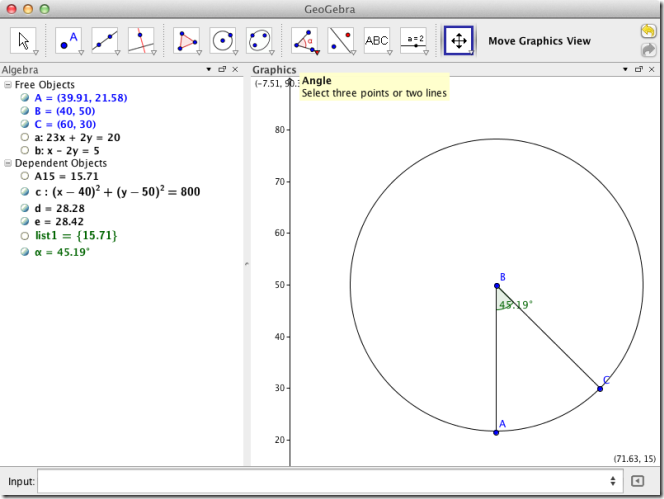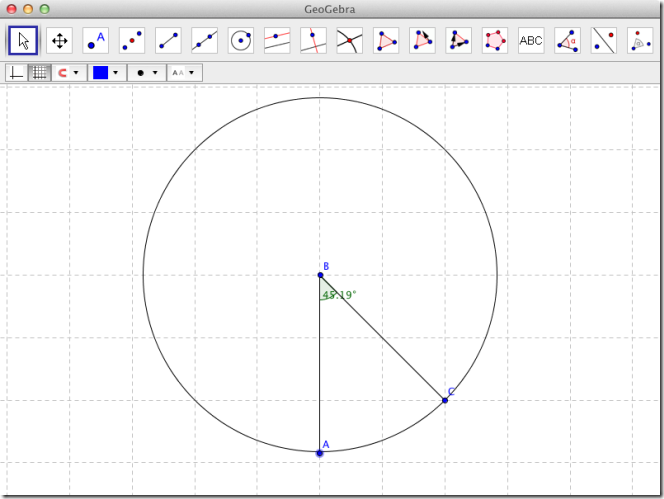# GeoGebra: Plot Algebraic Expressions, Find Geometric Coordinates [Mac]

If you hated Math and disliked your Algebra teacher with the force of a thousand Reddit downvotes, then you will envy GeoGebra. In all fairness, no one in their right mind would try and plot algebraic equations unless it were required. You seldom hear people say, “I’m going to chill out with this geometric theorem”, but for those that are still doing sums and plotting lines, this app is heaven sent. GeoGebra is a Mac app that plots algebraic equations for you. It also lets you place any two points on a graph and find out the resulting equation, plot angles & geometric shapes, and find their coordinates. Life would indeed have been easier if this app were allowed in school.

The app has four different views, and all views (called perspectives) are interlinked; that is, the variables entered in one view are remembered and can be referenced when working in another one. The four views are Algebra & Graphics, Basic Geometry, Geometry, and Spreadsheet & Graphics.

The Algebra & Graphics view allows you to enter and get algebraic equations. To enter an equation, type it in the Input field at the very bottom. To plot any two random points, use the buttons at the top. The New Point button allows you to place any number of points on the graph, and the Line through two points button lets you connect two points. As you enter and connect points, the panel on the left displays the coordinates of all points and any equations that are formed.Additional buttons at the top allow you to create polygons and circles, create an angle, and more. If you feel this isn’t quiet what you need, you can always switch over to the simple geometric view, and plot the figure you want, enter points that you want coordinates of, and create angles wherever needed. Return to the algebraic view and equations and points will have been detailed for you.The spreadsheet view allows you to enter statistical formulae and conduct a one-variable analysis. The app, with its simplicity and comprehensive set of features is perhaps a dream come true for people plagued with sums and figures.

1.Guest says:
2.Guest says: